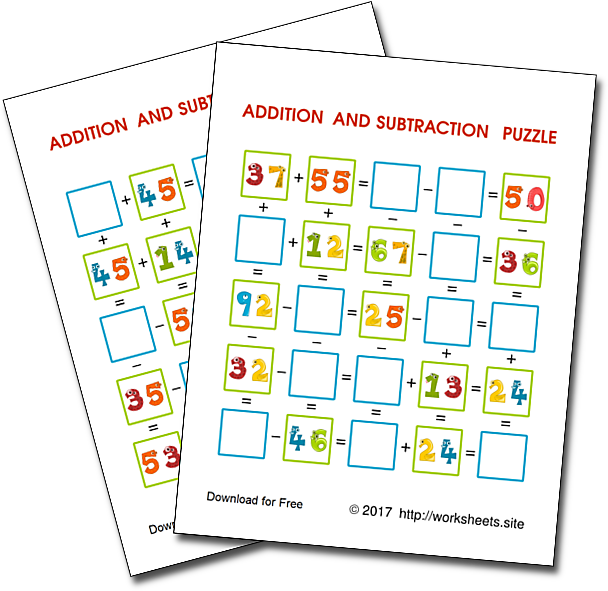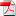Practice Sums and Differences with a fun game. Solve the math puzzle by doing sums and differences. Games for working with additions and subtractions. Free printable worksheets to practice addition. Fun math worksheets to learn math operations for free. Pastimes and activities for 4th to 6th graders.How to practice sums in a fun way? Practice what you've already learned. Fill the empty spaces is the grid with the numbers that make the addition and subtraction operations true. Use these colorful games to improve your math skills and fight boredome at the same time!

These exercises allow students to practice addition and subtraction of whole numbers with two-digit numbers. Children fill the empty boxes using the clues in the other boxes.

The students will to use their reasoning to complete some squares, for example in an operation of the type: ? + 3 = 7, the student must make subtraction to fill the square with a 4. For this reason, these exercises are useful as a preparation for the Algebra, since they show the unknowns as empty boxes, as it usually happens in books of pre-algebra and also shows the usefulness of the concept of equation and resolution of an equation.

Since each grid has many clues, the students could check if they have correctly filled-in a square, using the information of several clues, some horizontal and others vertical. For example: if the horizontal clue was ? + 3 = 7, that same empty square could be filled with a vertical clue, 9 - 5 = ?. If the student writes 4, one clue was used as the question and the other clue as a verification. Being able to have the means to know if an answer is right or wrong quickly, without explicitly revealing what the answer is, makes learning easy.

Each worksheet contains a game or puzzle. The document contains 30 pages, with two-digit additions and subtractions. It is in PDFformat. Use the password worksheets.site to open the PDF file. This is a pencil and paper activity that does not require any cutting or gluing.

## 1. How to use

These games can be used in a variety of scenarios, to practice two-digit sums and differences:

Homework

These worksheets are ideal for homework. They are a fun alternative to regular homework and parents can help their children along, however children can guide and self-correct themselves by checking whether all the clue answers agree with each other. It is very popular with children and parents.

Who finishes first

You can hand identical sheets out to every student in the class and do a speed race, with prizes, for students who complete the whole puzzle first.

Complementary Activities

The game is a perfect activity for those children who finish their work early and are left with nothing to do. It can be printed and kept in a game box or on a shelf where children can access it when they finish their work.

Peer Teaching

These printable games are a great activity that students can use to help others. Pair a slower student with a faster student so that students can teach each other.

Students as Teachers

An activity that is really worthwhile is that some children learn the game and then teach others. Teaching others how to play is a great communication activity.